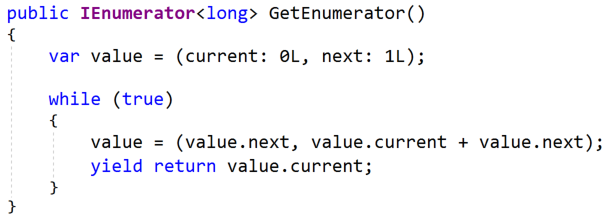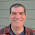## Tuesday, April 25, 2017

### Implementing a Fibonacci Sequence with Value Tuples in C# 7

I decided to do some experimentation with the Fibonacci Sequence. I wanted to see if I could implement it with value tuples in C# similar to the way that I had previously done with F#. And I wanted to see how similar or different they are.

Previously, I implemented a Fibonacci Sequence in C# by TDDing into the code. The code is pretty imperative, but the readability is okay (it could be better):

Even better, we have a set of passing unit tests. So we can safely do experimentation and know when we've messed something up.

Note: you can grab the code for this article on GitHub: jeremybytes/fibonacci-tdd. Both the TDD and value tuple steps are included as branches.

The Idea (from F#)
Here's the idea: last year I worked through the first 10 Euler problems to learn F# a bit better. In particular, Euler #2 uses the Fibonacci Sequence. The implementation that I ended up with used tuples, and I wondered if I could use the C# 7 value tuples to create something similar.

Here's the code from the F# implementation (you can see the whole thing here: Functional Practice: Euler Problem #2 in F#):

This uses a "fold" which runs the specified function for each item in the list and hangs on to an accumulator value. In this case, the accumulator is a tuple that is initialized to "(0,1)". Then for each item in the list, it will create a new tuple consisting of the second value of the original ("b") and the sum of the first and second values ("a + b"). For a deeper dive, check out that article.

My implementation in C# will be a little bit different from this. First, this function gets the "nth" Fibonacci value. So if I call this function "fibonacci 4", it will give me the single value of "3". Instead of returning the "nth" value, we want to create a sequence that iterates through all the values (and yes, the F# article does describe that a little later, but we'll take things one step at a time).

Switching to Value Tuples
Rather than using a "fold" (or the LINQ version "Aggregate"), we'll stick with the "while" loop to return the values as they are requested. But we still want to use the same type of tuple for the accumulator.

So the first step is to create a tuple variable that we can initialize to "(0,1)":

As mentioned in a prior article, we cannot simply use a value tuple out of the box (even though we're using Visual Studio 2017 and C# 7). The feature has to be brought in through a NuGet package. I'm still not happy about how this works today. As Thomas Levesque pointed out in the comments, the release order is kind of out of whack. Welcome to the new world.

Anyway, we just need to bring in the "System.ValueTuple" package:

And everything works:

One slight difference is that we have the initial values noted as "0L" and "1L". This tells the compiler that we want to use the "long" integer type, and it will create the correct tuple with 2 long values.

Now we just need to do the same math as in the F# function and return the result:

Just like the F# "fold" function above, we create a new tuple where the first element is the second element of the previous value, and the second element is the sum of the first and second elements.

Then we return the first element of the tuple (which is what the "fst" function does in the F# code).

The good news is that all of the tests still pass:

Great! That means we have a working implementation.

The readability of this is not great. One thing we can do is give our tuple elements names. We'll use "current" and "next":

This makes it a little bit easier to keep track of the elements in our tuple. And it's also clear that we're returning the correct value "current".

And importantly, all of our tests still pass:

Is This Better?
It's great that I got to experiment some with value tuples (and sad that I ran into the issue trying to use them initially). But is this a better implementation?

Let's look at them side-by-side:Original ImplementationWith Tuples

Neither of these implementations is particularly readable. The original version suffers because we're using the previous, current, and next variables and swapping them from place to place. It's a bit confusing to figure out exactly what's happening.

The tuple version has kind of the same problem. We have eliminated one of the variables so now we only have two (current and next), but we're still doing a swap and math that isn't real clear.

Wrap Up
The fun thing about experimenting is that you're never quite sure what you'll find. In this case, it's interesting that we can implement the Fibonacci Sequence fairly easily using value tuples. But I don't think we've improved readability much. Less code is often good, but not if it obfuscates things.

I think I'd have to call these implementations a "draw" in their current state. They both have similar readability issues. But that just means that we can do some more experimentation to see what we can come up with.

Happy Coding!

1.Would you consider the following more readable? It eliminates the value variable.

public IEnumerator GetEnumerator() {
long current = 0;
long next = 1;
while (true) {
(current, next) = (next, current + next);
yield return current;
}
}

1.This is interesting. My initial reactions are mixed. (But my reactions for the implementations in this article are mixed as well.). I'm going to have to ponder it for a bit. Thank you for your solution. Folks sharing their code has let me think about things in different ways, and I know I'm a lot better because of that.